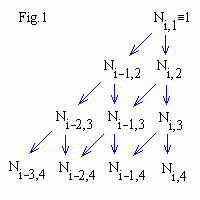# B-spline basis functions

The equation for k-order B-spline with n+1 control points (P0 , P1 , ... , Pn ) is
P(t) = ∑i=0,n Ni,k(t) Pi ,     tk-1 ≤ t ≤ tn+1 .
In a B-spline each control point is associated with a basis function Ni,k which is given by the recurrence relations (see also b-spline.js)
Ni,k(t) = Ni,k-1(t) (t - ti )/(ti+k-1 - ti ) + Ni+1,k-1(t) (ti+k - t)/(ti+k - ti+1 ) ,
Ni,1 = {1   if   ti ≤ t ≤ ti+1 ,    0   otherwise }

Ni,k is a polynomial of order k (degree k-1) on each interval ti < t < ti+1. k must be at least 2 (linear) and can be not more, than n+1 (the number of control points). A knot vector (t0 , t1 , ... , tn+k ) must be specified. Across the knots basis functions are C k-2 continuous.Corresponding iterations scheme for cubic (k = 4) basis functions is shown in Fig.1 . You see, that for a given t value only k basis functions are non zero, therefore B-spline depends on k nearest control points at any point t.

The B-spline basis functions as like as Bezier ones are nonnegative Ni,k ≥ 0 and have "partition of unity" property
i=0,n Ni,k(t) = 1,     tk-1 ≤ t ≤ tn+1
therefore
0 ≤ Ni,k ≤ 1.
As since Ni,k = 0 for t ≤ ti or t ≥ ti+k therefore a control point Pi influences the curve only for ti < t < ti+k .

## Knot vectors

The shapes of the Ni,k basis functions are determined entirely by the relative spacing between the knots (t0 , t1 , ... , tn+k ). Scaling or translating the knot vector has no effect on shapes of basis functions and B-spline. Knot vectors are generally: uniform, open uniform and non-uniform.
Uniform knot vectors are the vectors for which   ti+1 - ti = const,   e.g. [0,1,2,3,4,5].
Open Uniform knot vectors are uniform knot vectors which have k-equal knot values at each end:
ti = t0 ,   i < k
ti+1 - ti = const ,   k-1 ≤ i < n+1
ti = tk+n ,   i ≥ n+1

e.g. [0,0,0,1,2,3,4,4,4]   (k=3, n=5).
Non-uniform knot vectors. This is the general case, the only constraint is the standard ti ≤ ti+1 .

## The main properties of B-splines

• composed of (n-k+2) Bezier curves of k-order joined C k-2 continuously at knot values (t0 , t1 , ... , tn+k )
• each point affected by k control points
• each control point affected k segments
• inside convex hull
• affine invariance
• uniform B-splines don't interpolate deBoor control points (P0 , P1 , ... , Pn )

## Uniform B-splines

The principle thing to note about the uniform basis functions is that, for a given order k, they are simply shifted copies of one another (because all the knots are equispaced). You can see below, that increasing the order k increases smoothness of a curve and tends to move the curve farther from its control polygon.

## Linear B-spline (n = 3, k = 2)

In the right window you see basis polynomials. Move a knot to see how it influences on spline shape and basis functions. B-spline curve is composed of (n-k+2) segments painted in different colors. Corresponding t intervals (in the right window) are painted in the same colors.

Interactive B-spline   Use finger or mouse to move a nearest control point (a small blue square in the left window) or knot (a small black square in the right window).

## Closed curves (n = 5, k = 4)

To make a C k-2 continuous closed loop you need only, that the last k - 1 control points repeat the first k - 1 ones, i.e. [P0 , P1 , P2 , P0 , P1 , P2 ] for n = 5, k = 4 (in this example the last 3 points are displaced a bit to make them visible).
Contents   Previous: Building cubic B-spline   Next: Nonuniform B-splines
updated 8 August 2001